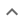•# Simplifying square roots

We think you wrote:

###This solution deals with simplifying square roots.

Solution found3a*sqrt(2a)

## Step by Step Solution## Step  1  :

#### Simplify the Integer part of the SQRT

Factor 18 into its prime factors
18 = 2 • 32

To simplify a square root, we extract factors which are squares, i.e., factors that are raised to an even exponent.

Factors which will be extracted are :
9 = 32

Factors which will remain inside the root are :
2 = 2

To complete this part of the simplification we take the squre root of the factors which are to be extracted. We do this by dividing their exponents by 2 :
3 = 3

At the end of this step the partly simplified SQRT looks like this:
3 • sqrt (2a3)

## Step  2  :

#### Simplify the Variable part of the SQRT

Rules for simplifing variables which may be raised to a power:

(1) variables with no exponent stay inside the radical
(2) variables raised to power 1 or (-1) stay inside the radical
(3) variables raised to an even exponent: Half the exponent taken out, nothing remains inside the radical. examples:
(3.1) sqrt(x8)=x4
(3.2) sqrt(x-6)=x-3

(4) variables raised to an odd exponent which is  >2  or  <(-2) , examples:
(4.1) sqrt(x5)=x2•sqrt(x)
(4.2) sqrt(x-7)=x-3•sqrt(x-1)

Applying these rules to our case we find out that

SQRT(a3) = a • SQRT(a)

#### Combine both simplifications

sqrt (18a3) =
3 a • sqrt(2a)

## Simplified Root :

3 a • sqrt(2a)